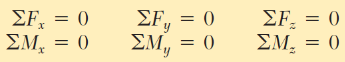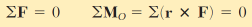Courses

# Equilibrium in 3D Mechanical Engineering Notes | EduRev

## Mechanical Engineering : Equilibrium in 3D Mechanical Engineering Notes | EduRev

The document Equilibrium in 3D Mechanical Engineering Notes | EduRev is a part of the Mechanical Engineering Course Engineering Mechanics - Notes, Videos, MCQs & PPTs.
All you need of Mechanical Engineering at this link: Mechanical Engineering

Equilibrium in Three Dimensions

Six scalar equations are required to express the conditions for the equilibrium of a rigid body in the general three-dimensional case, those equations are listed below:These equations can be solved for no more than six unknowns, which generally will represent reactions at supports or connections.

During solving the most of the problems, the above scalar equations will be more conveniently obtained if we first expressed in vector form the conditions for the equilibrium of the rigid body considered.

We write and express the forces ‘F’ and position vectors ‘r’ regarding scalar components and unit vectors. Next, we compute all vector products, either by direct calculation or using determinants.We observe that as many as three unknown reaction components may be eliminated from these computations through a judicious choice of the point ‘O’. By equating to zero the coefficients of the unit vectors in each of the two relations (see the above equation), we obtain the desired scalar equations.

Offer running on EduRev: Apply code STAYHOME200 to get INR 200 off on our premium plan EduRev Infinity!

,

,

,

,

,

,

,

,

,

,

,

,

,

,

,

,

,

,

,

,

,

;